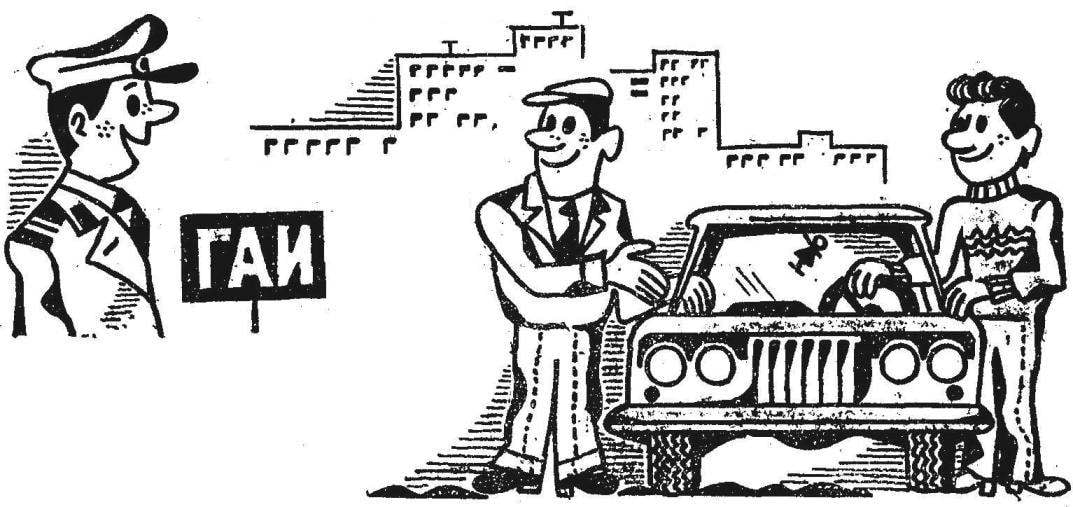# BUILDING A CAR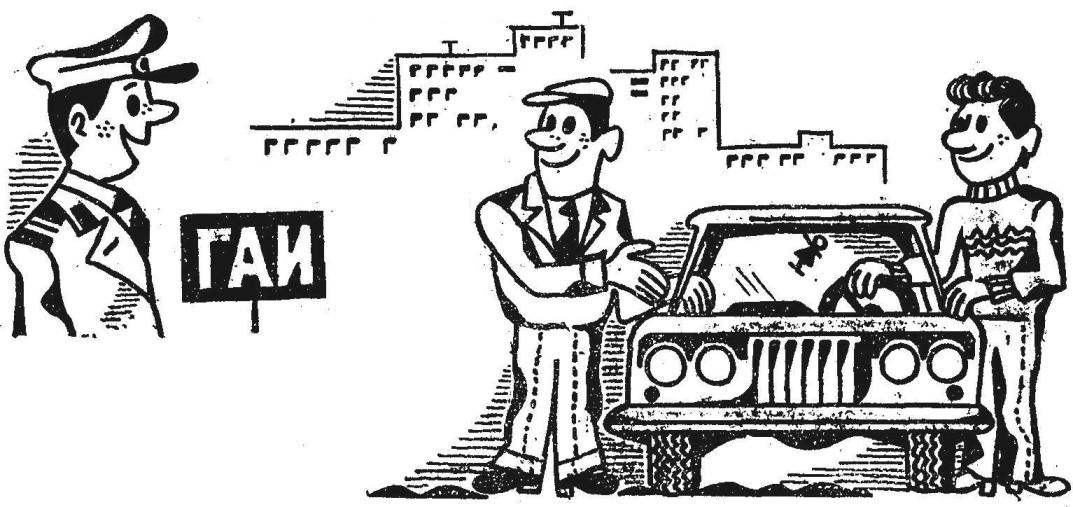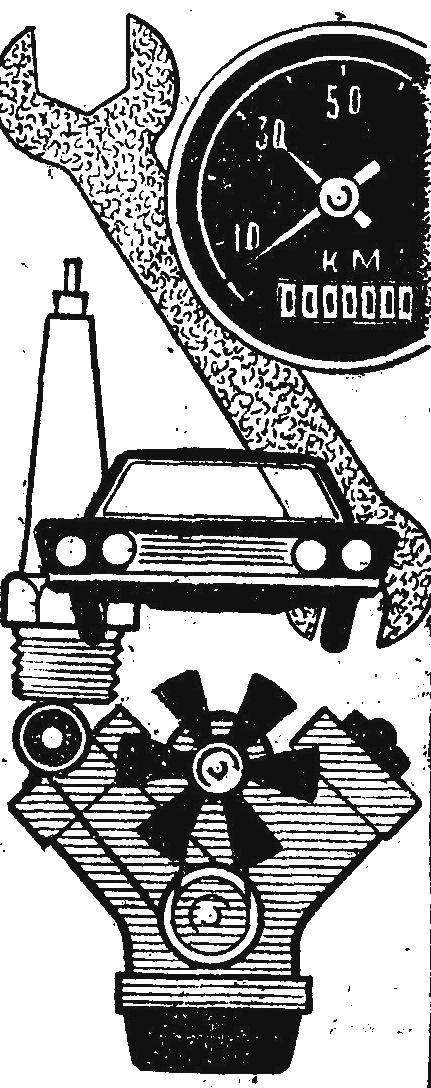Amateur designers often begin construction of the car, relying only on intuition, without any calculations. Most often in such cases the performance of the machine be not the such of any wanted the Creator, and the individual components break or wear out quickly.

To minimize “Surprises” from pre-calculations. The most essential of them under the force of each Builder of the car. The individual responsible for the details of the calculations are given directly in the description. Here we will talk only about the main associated with common structure and characteristics of the car, just as we in a previous publication estimated the mass distribution.

HOW TO ENSURE THE FULLEST USE OF THE POWER AND TORQUE OF THE ENGINE? For this you need to choose the gear ratios of the transmission. Transmission, typically uses, together with the engine; its characteristics are determined. It is not always possible to purchase tyres of the desired size; but, in any case, the diameter is known. Thus, the case boils down to calculating the gear ratios of the main transmission axle. We present it in a simplified form.

He begins with the definition of the maximum speed, which is capable of designing a car with this engine. There are two possible options for calculating the to reach the greatest possible speed or perhaps acceleration, is more easily overcome the climbs and bad roads.

It should be noted that in the calculation according to the second embodiment, the highest speed obtained is slightly less than on the first.

TABLE 1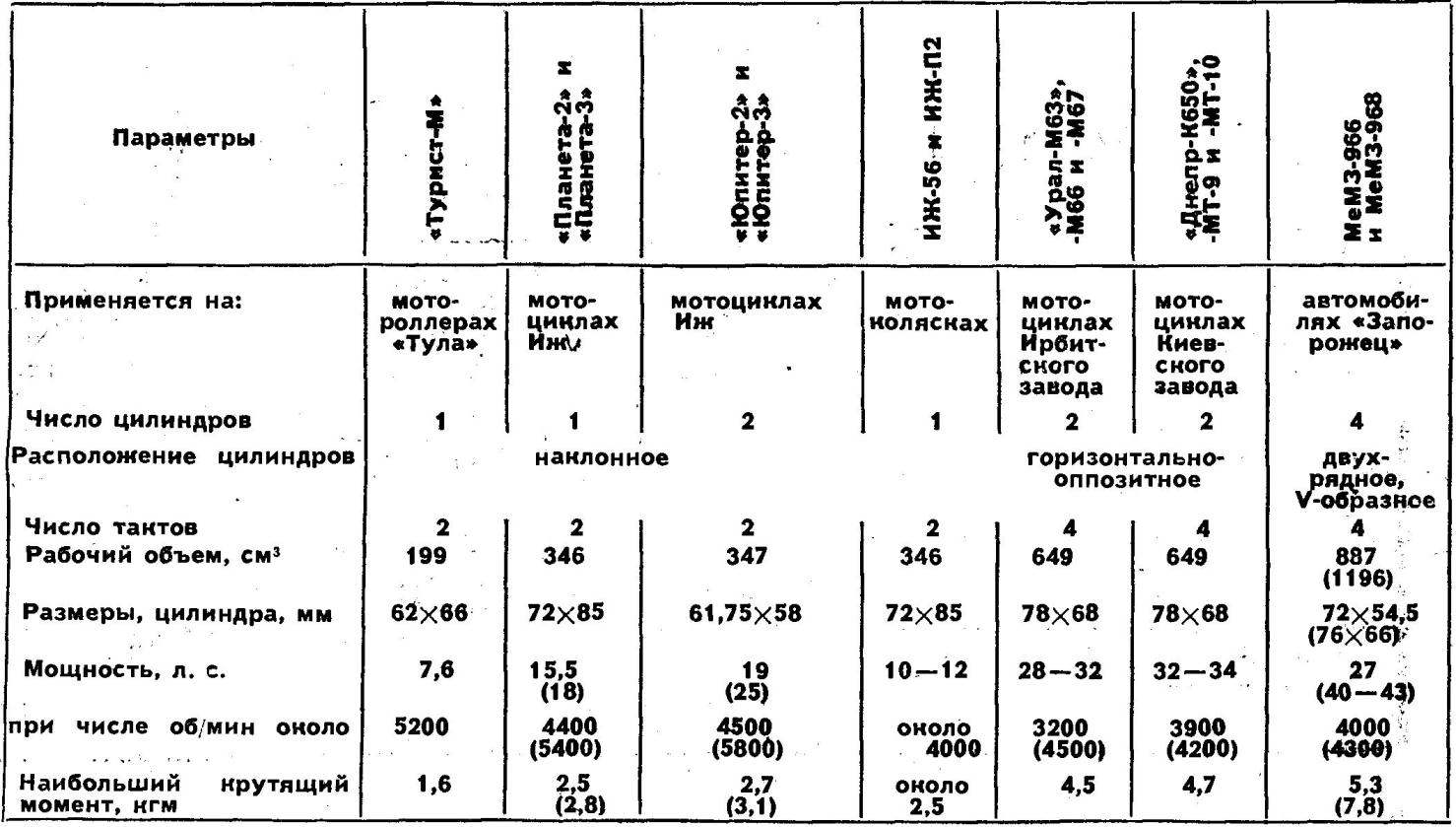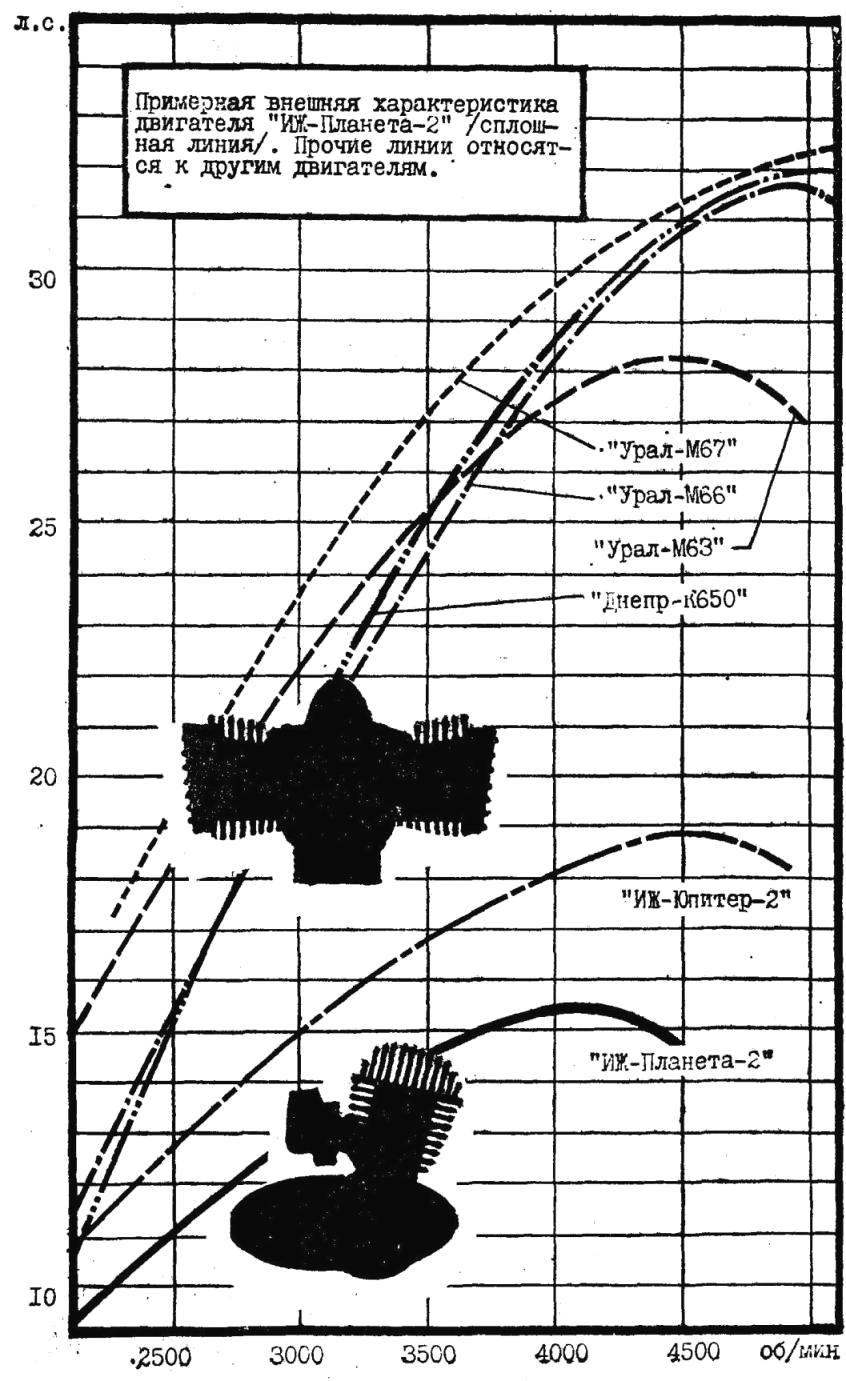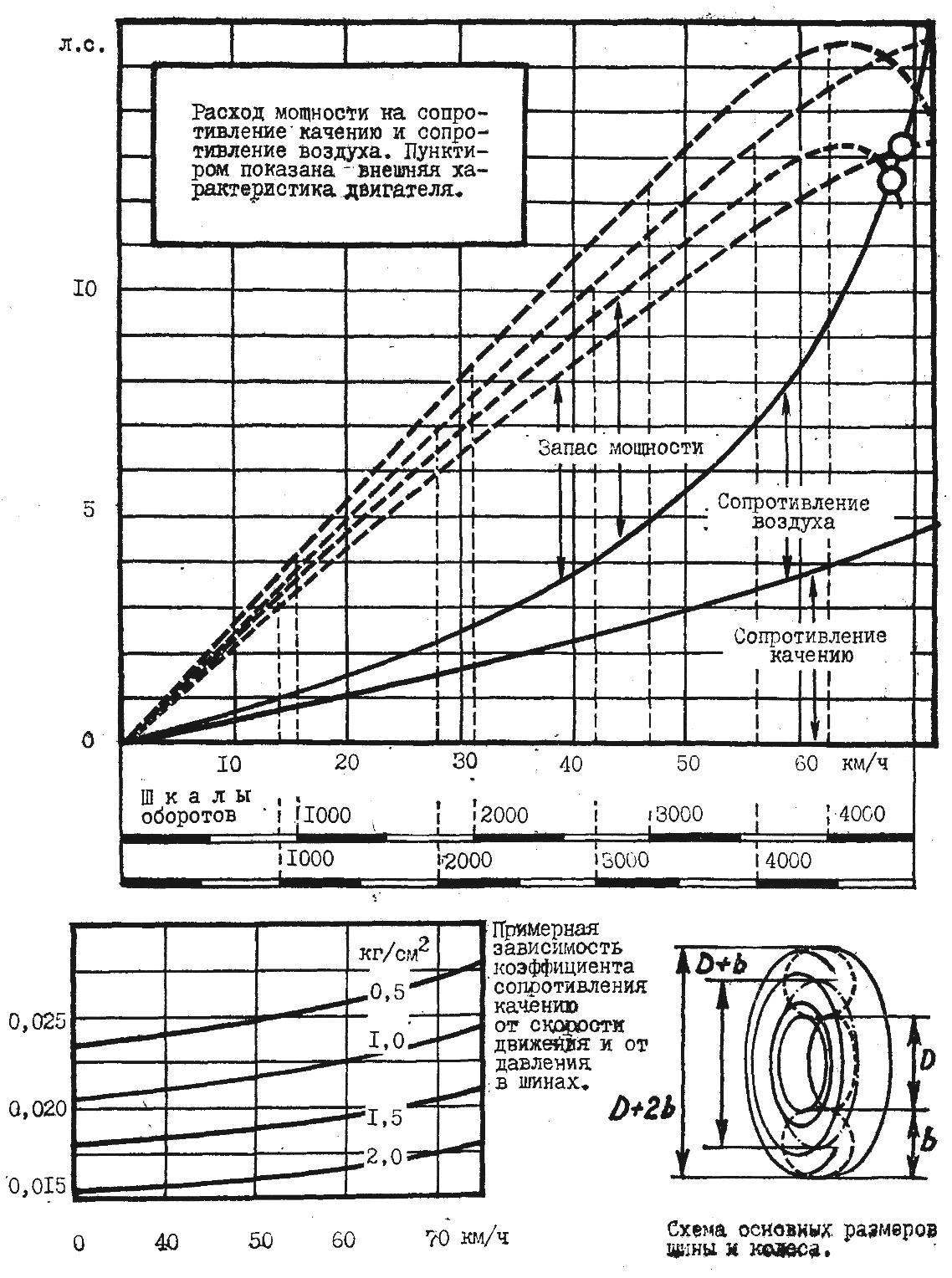In the first case, based on the fact that the maximum speed the car reaches at the moment when the engine develop highest efficiency; the second — at the maximum permissible number of revolutions. In most engines it is on 10 — 15% higher than the rpm of maximum power; power is thus correspondingly reduced; as they say, it is “behind the bend”.

Take for example the engine of a motorcycle “IZH-Planeta-2”, which is developing at 4100-4600 rpm 15,5 HP, or about 12 kW for a new measurement system (see graph and table. 1) where are also brief information on other engines). For simplicity we will not take into account the reduction in engine output in connection with the possible application fan forced cooling. The engine is scheduled to be installed in a four-seater four-wheeled vehicle with a light open body. Design full weight Gа car (with load 300 kg) is 800 kg. the Coefficient of air resistance for a car is equal to about 0.05; frontal area F, about 1.5 m2. Used from Serpukhov sidecar tire size 5.00—10 internal pressure should be an average of about 1.3 kg/cm2 (calculation of the tire pressure given below). The η coefficient of performance (COP) of the Transmission taking into account the possible additional gear or chain transmission is assumed equal to 0,85.

Build a graph of the power consumption required for rolling resistance (Nf) and air resistance (Nw), depending on the speed Va of the vehicle. Power is calculate by the formula:

Nf = (Ga·f·Va)/270 (HP)

Nw = (K·F·Va3)/3500 (HP)

The rolling resistance coefficient f is a variable; it can be determined with sufficient accuracy in the third schedule.

As you can see, the calculation in the first embodiment, that is, when power to the drive wheels, is equal to 15,5X0,85 — 13 HP, you can expect the car has reached a speed of about 70 km/h. When calculating according to a second embodiment of the power will be about 12 HP, with a speed of a little over 65 km/h.Ask the car speed of 65 — 70 km/h or more — not to count on frequent movement. It may sound strange, but we argue that some high max speed is needed to… safety. The fact that overtaking is even wheelchair sidecar or moped going at a speed of 40 km/h, overtaking requires a speed of at least 50 km/h To be overtaking was “energetic”, safe, and the road cleared quickly, the drivers typically include a third gear in which it is possible to travel at a speed approximately 25 % smaller than the maximum (see table. 2).

TABLE 2. THE VALUES OF THE TRACTION FORCE AND SPEED IN DIFFERENT GEARS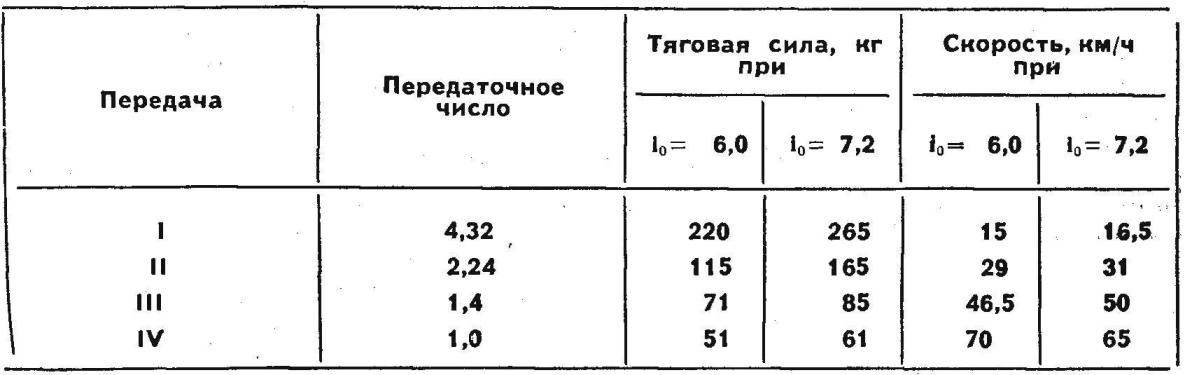Receiving data about the maximum speed you can set the gear ratio i of theo of the main transmission by the formula:

io = (0,377·rk·n)/Va

In this formula, CC is the rolling radius of the wheel equal to (for wheels 5.00—10) 0.25 m, and n is the number of revolutions of the engine per minute: 4500 to calculate according to the first embodiment, and 5,000 for the second. Gear ratio respectively of 6.0 and 7.2.

We should not confuse large values of these numbers as compared to those for the mass passenger cars: powertrain motorized wheelchairs are available, in addition to the main transmission, for two chain with a total gear ratio around 2.75, so that the main transmission remains a 2.2 or 2.6 (instead of 2.08 from sidecar).

Recall that the calculation we carry out, keeping in mind the use of standard gear box sidecar, which has a gear ratio of the fourth (highest) transmission equal to one. For calculation of the cars of the closed type, the coefficient K is equal to about 0.04, for a well-streamlined car — about 0.03. Driveline efficiency in the absence of the double gear or chain transmission should be taken equal to 0,9.

Now let’s check the results of our calculation. Superimpose on the graph the power consumption (dotted lines) graph external characteristics of the engine. To build it under the scale speed scale speed motor, and depending on the adopted variant of the calculation of the superposed mark on the scale 4500 rpm with a mark of 70 on a scale of speed or level 5000 with a mark of 65, and then divide the resulting segments 0-4500 or 0-5000 marks at 500 rpm, respectively, at the 9 or 10 parts. Restoring from a stamp on one of the new scales vertical chart, the external characteristics of the engine, put on them the values of power reduced by 15% (h = 0,85) compared to the values of the source characteristics. We see that the car is calculated according to the second embodiment, should have plenty of power and is therefore relatively well to take acceleration and climbs.

Whether there is sufficient tractive force RK for movement design of the car in different conditions? It is calculated by the formula:

Pk = (Me·io·ik·η)/rk (kg)

In this formula, Me — the maximum torque of the engine (in our case it is equal to 2.5 KGM), andK is the gear ratio in the gearbox.

Substituting the value of Ion (for 6.0 or 7.2) and itothe received values of the traction force in kg (table. 2).

For car traffic it is necessary that the traction force RK to the drive wheels was less than the force of adhesion of wheels with the ground (otherwise the wheel will slide, to slip) and not less than the resistance force. Adhesive force equals the mass of the vehicle attributable to the drive wheels, the adhesion factor (of 0.7 for dry pavement, 0.15 and wet). On the rear wheels of our car have mass, equal to about 60% of the total mass, i.e., 800 X 0.6 = 480 kg. Thus, the traction on dry pavement is equal to 335 kg, wet 51 kg. the Force of resistance to motion equals the mass of the car on the rolling resistance coefficient f, which on the asphalt (see chart) is equal to about 0.02, on a country road — 0,035, the sand is about 0.2. Here are respectively 16, 28 and 160 kg.

So, the designed car will perform well on dry pavement. But to move in first gear need, not giving full acceleration. On a slippery road will also need to reduce pressure on the accelerator pedal, so as not to cause slipping of the wheels. On country roads the car will go in all gears, but the sand will have to switch to the second (the second variant of calculation) or even on first gear (the first option).

Thus, we can conclude that the second option is expensive, because the difference in maximum speed is small, the possibility of the car to overcome the difficult sections of the road (and lifts) at a higher gear ratio is much better.

Very important the correct choice of tire and internal pressure. And for that, there are also simple calculations.

Builders of home-made vehicles can rely on the use of tyres from scooters, motorized wheelchairs, from the car “Zaporozhets”, “Lada” and “Moskvich” (on the 13-inch or 330-mm rims).

The value of the required internal pressure p of the load or the value of the permissible load G. when the desired internal pressure can be roughly calculated according to the formulas:

Gk = (5b2·(D+b)·(p+1))/(D+2b) (kg)

p = (Gk·(D+2b))/(5b2·(D+b))-1 (kg/cm2)

In these formulas D is the diameter of the rim, and b is the section width, expressed in inches (see diagram), as it was adopted and preserved to this day in the naming of tyres. Formulas are based on the assumption that the quantity of compressed air contained in the tire should always have the same number of kilograms of load. Substituting letters are known or selected values for the size of tire and pressure, we can calculate the values of load (and Vice versa).

For example, in the above case, the Builder has a tire size 5.00—10; weight of vehicle with load — 800 kg, of which 480 kg have on the rear wheels, that is equal to 480 :2 = 240 kg, then:

p = (240·(10+10))/(5·25·(10+5))-1 = 1,5 (rounded)

For the front tires the same way get a 0.7.

Almost pressure take 2-3 tenths kg/cm2 more than the resulting calculation (in our case, the 1.7 and 0.9) to reduce rolling resistance and prolong tire life. However, for smooth running little car it is desirable that the tire pressure was as low as possible, especially in the tyres of the wheels, close to which a seat (for example, for the removal of the driver’s seat forward).

A definite relation between the units of pressure in the front and rear tires and significantly more to vehicle stability, one of the characteristic indicators of which the value of the so-called critical speed Vpx, calculated approximately according to the equation: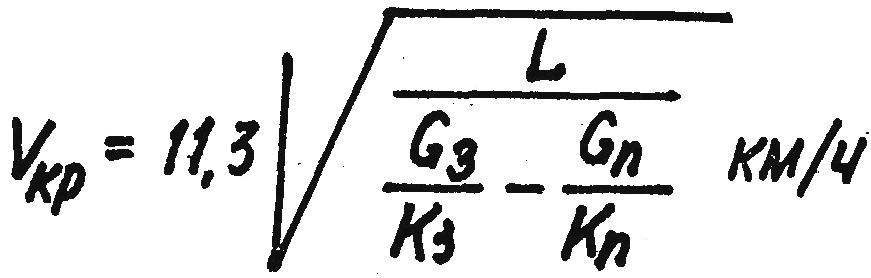In this equation: L is the base of the car in m, G3 and Gп — load accordingly on the rear and front wheels in kg, K3 and KP— coefficients of resistance to slip of tyres (two for each axis). The last coefficients are calculated according to the equation:

K = 5b·(D+2b)·(p+1)

The coefficient K shows a lateral force in kg is required to rotate the wheel to an angle equal to one radian (53 °).

Take for example the same car with the pressure in the front tires of 0.9 kg/cm2, at the rear of 1.7 kg/cm2 and a base of 2 m. the Coefficients of resistance to withdrawal of the tire will be:

K3 = 5·5·(10+10)·(1,7+1) = 1350 kg/rad

Kn = 5·5·(10+10)·(0,9+1) = 950 kg/rad

Then: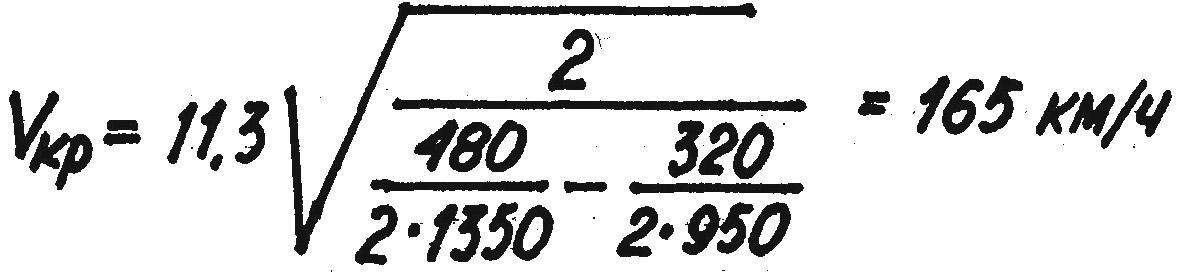that is, more developed by the vehicle speed. This is required for vehicle stability. If the critical speed was less than maximum, would have to reduce the pressure in the front tires (if applicable) or increase — in the back.

Information on tires given in table 3.

TABLE 3. BRIEF DESCRIPTION OF THE TIRE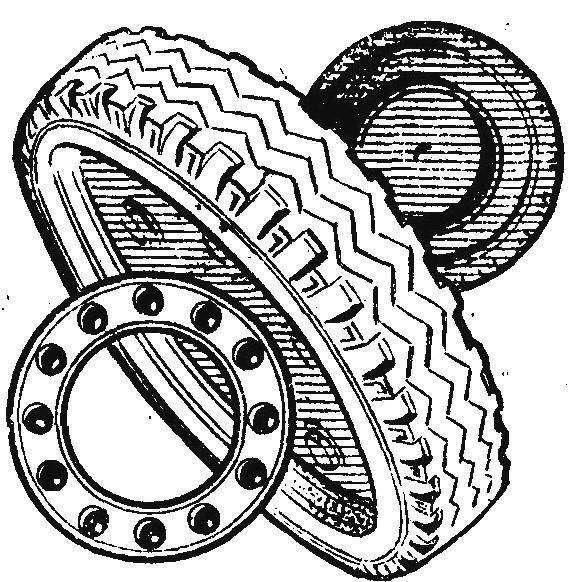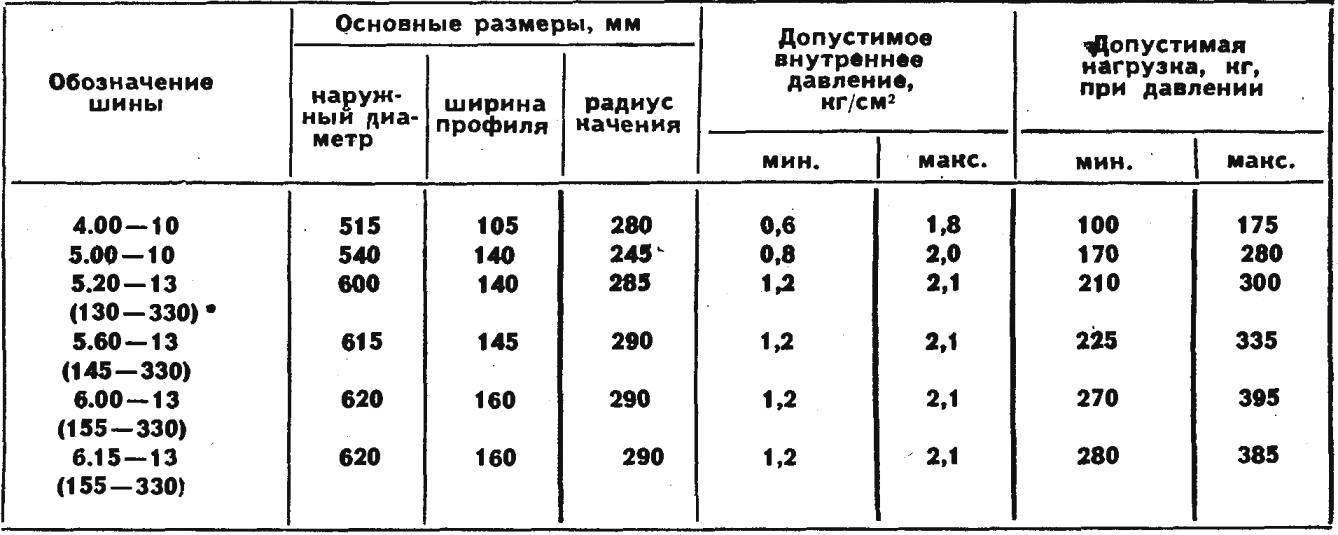These are the most necessary calculations that need to produce to obtain a satisfactory overall performance of a homemade car.

Y. DOLMATOVSKY, candidate of technical Sciences